# Blue Noise Dithering

## Initial seed pattern

This is a short description on blue noise dithering. For more detail see the 1993 paper about blue noise dithering.

Initially a binary pattern BP is created where N pixels (about 10 percent) are set to 1 and the rest to 0.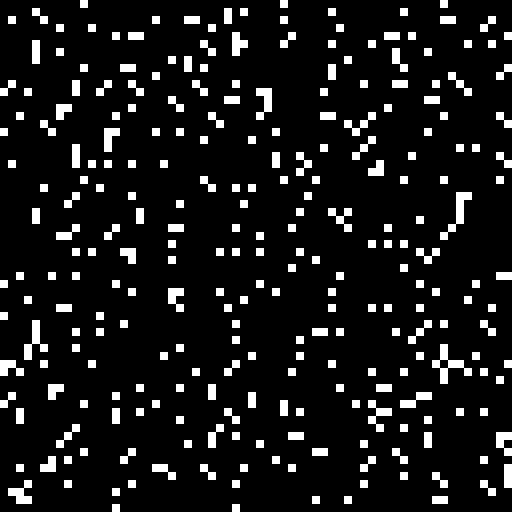The binary pattern then is convolved with the following filter function to generate the density array DA:You can use a sigma value of 1.5.

The convolution is wrapped around to facilitate a tileable result: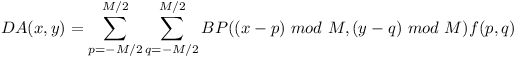Maxima of the density array are called clusters and minima are called voids.

The 1 value in BP with the highest density value DA (tightest cluster) is set to 0 and DA is updated accordingly. Now the 0 value in BP with the lowest density value DA (largest void) is set to 1 (and DA is updated).

This is repeated until disolving the tightest cluster creates the largest void. This is done to spread the 1 values evenly.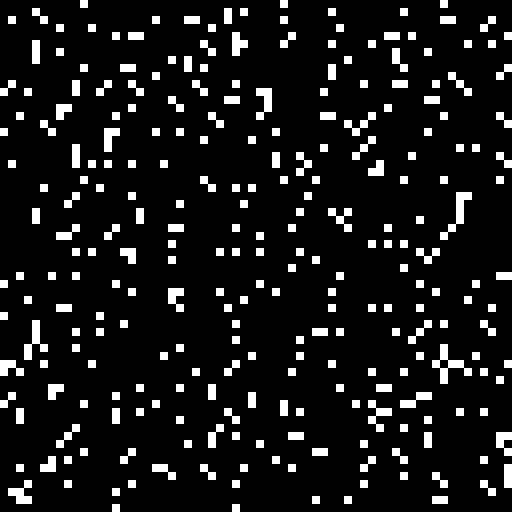## Phase 1

In phase 1 of the dithering algorithm the 1 values of a copy of the seed pattern are removed one by one starting where the density DA is the highest. A copy of the density array DA is updated accordingly. The corresponding positions in the resulting dither array are set to N-1, N-2, …, 0.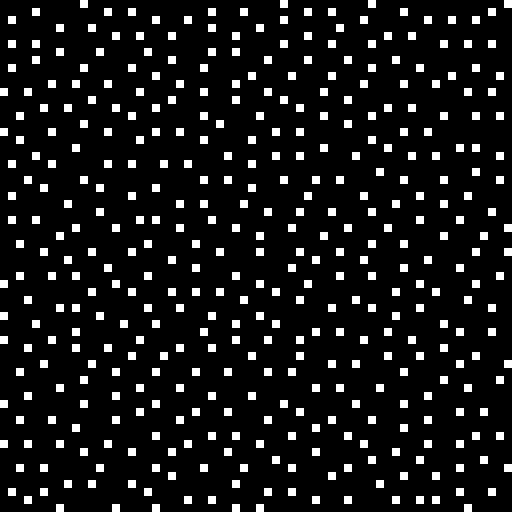## Phase 2

In phase 2 starting with the seed pattern a mask is filled with 1 values where the density DA is the lowest. The density array DA is updated while filling in 1 values. Phase 2 stops when half of the values in the mask are 1. The corresponding positions in the dither array are set to N, N+1, …, (M * M) / 2 - 1## Phase 3

In phase 3 the density array DA is recomputed using the boolean negated mask from the previous phase (0 becomes 1 and 1 becomes 0). Now the mask is filled with 1 values where the density DA is the highest (clusters of 0s) always updating DA. Phase 3 stops when all the values in the mask are 1. The corresponding positions in the dither array are set to (M * M) / 2, …, M * M - 1.## Result

The result can be normalised to 0 to 255 in order to inspect it. The blue noise dither array looks as follows:Here is an example with constant offsets when sampling 3D clouds without dithering.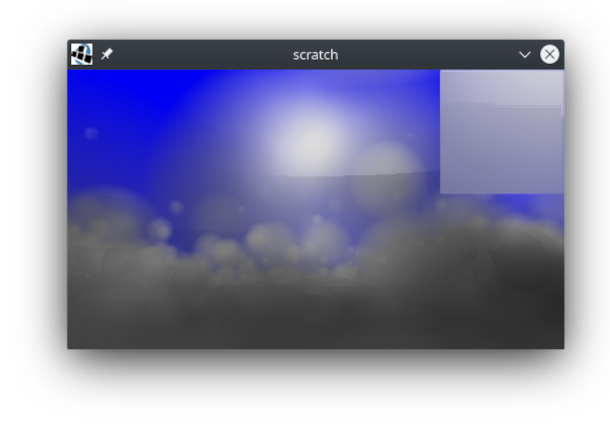Here is the same scene using dithering to set the sampling offsets.One can apply a blur filter to reduce the noise.Note how the blurred image shows more detail than the image with constant offsets even though the sampling rate is the same.